# NCERT Solutions for Class 12 Physics Chapter 11 Dual Nature of Radiation and Matter

In this chapter, we provide NCERT Solutions for Class 12 Physics Chapter 11 Dual Nature of Radiation and Matter for English medium students, Which will very helpful for every student in their exams. Students can download the latest NCERT Solutions for Class 12 Physics Chapter 11 Dual Nature of Radiation and Matter pdf, free NCERT solutions for Class 12 Physics Chapter 11 Dual Nature of Radiation and Matter book pdf download. Now you will get step by step solution to each question. Class 12 physics is a very important subject for entrance exams like IIT JEE, CPMT, etc.

## NCERT Solutions for Class 12 Physics Chapter 11 Dual Nature Of Radiation And Matter

NCERT Exercises

Question 1.
Find the:
(a) maximum frequency, and
(b) minimum wavelength of X-rays produced by 30 kV electrons.
Solution:
(a) Maximum energy of X-ray photon = Maximum energy of an accelerated electron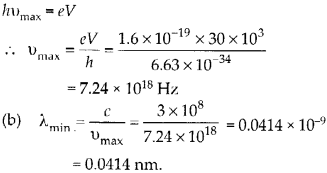Question 2.
The work function of caesium metal is 2.14 eV. When light of frequency 6 × 1014 Hz is incident on the metal surface, photo emission of electrons occurs. What is the:
(a) maximum kinetic energy of the emitted electrons,
(b) stopping potential, and
(c) maximum speed of the emitted photo-electrons?
Solution:

Question 3.
The photoelectric cut-off voltage in a certain experiment is 1.5 V. What is the maximum kinetic energy of photo electrons emitted?
Solution:

Question 4.
Monochromatic light of wavelength 632.8 nm is produced by a helium-neon laser. The power emitted is 9.42 mW.
(a) Find the energy and momentum of each photon in the light beam.
(b) How many photons per second, on the average, arrive at a target irradiated by this beam? (Assume the beam to have uniform cross-section which is less than the target area)
(c) How fast does a hydrogen atom have to travel in order to have the same momentum as that of the photon?
Solution: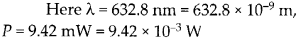(a) Energy of each photon,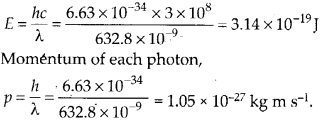(b) Number of photons arriving per second at the target,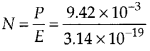(c) As mv = p
∴ Velocity,$\upsilon =\frac { p }{ m } =\frac { 6.63\times 10^{ -27 }kgms^{ -1 } }{ 1.67\times 10^{ -27 }kg }$
= 0.63 m s-1

Question 5.
The energy flux of sunlight reaching the surface of the earth is 1.388 × 103. How many photons (nearly) per square metre are incident on the earth per second? Assume that the photons in the sunlight have an average wavelength of 550 nm.
Solution:
Energy of each photon,Number of photons incident on earth’s surface per second per square metre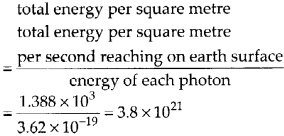Question 6.
In an experiment on photoelectric effect, the slope of the cut-off voltage versus frequency of incident light is found to be 4.12 × 10-15 V s. Calculate the value of Planck’s constant.
Solution: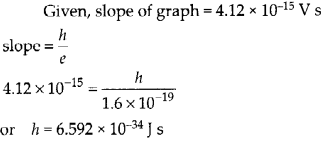Question 7.
A 100 W sodium lamp radiates energy uniformly in all directions. The lamp is located at the centre of a large sphere that absorbs all the sodium light which is incident on it. The wavelength of the sodium light is 589 nm.
(a) What is the energy per photon associated with the sodium light?
(b) At what rate are the photons delivered to the sphere?
Solution:
Here P = 100 W, λ = 589 nm = 589 × 10-9 m
(a) Energy of each photon,(b) Rate at which photons are delivered to sphere,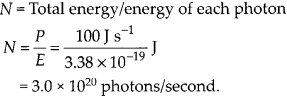Question 8.
The threshold frequency for a certain metal is 3.3 × 1014 Hz. If light of frequency 8.2 x 1014 Hz is incident on the metal, predict the cut off voltage for the photoelectric emission.
Solution:
According to Einstein’s relation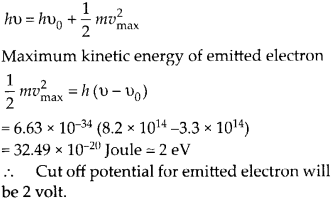Question 9.
The work function for a certain metal is 4.2 eV. Will this metal give photoelectric emission for incident radiation of wavelength 330 nm?
Solution:
Let us calculate the energy associated with photons incidentSince, energy of incident photons i.e., 3.77 eV is less than work function, hence no emission will take place.

Question 10.
Light of frequency 7.21 × 1014 Hz is incident on a,metal surface. Electrons with a maximum speed of 6.0 × 105 m s-1 are ejected from the surface. What is the threshold frequency for photo emission of electrons?
Solution:
According to Einstein’s equation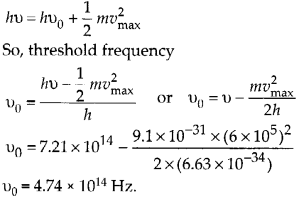Question 11.
Light of wavelength 488 nm is produced by an argon laser which is used in the photoelectric r effect. When light from this spectral line is incident on the emitter, the stopping (cut-off) potential of photo electrons is 0.38 V. Find the work function of the material from which the r emitter is made,
Solution: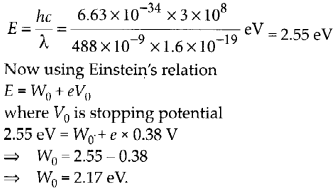Question 12.
Calculate the
(a) momentum, and
(b) de Broglie wavelength of the electrons accelerated through a potential difference of 56 V.
Solution:
An electron which is accelerated through a potential difference of 56 V will have kinetic energy K = 56 eV
(a) Momentum associated with accelerated electron(b) Wavelength of electron accelerated$\lambda =\frac { h }{ p } =\frac { 6.63\times 10^{ -34 } }{ 4.04\times 10^{ -24 } } =0.164 nm$

Question 13.
What is the
(a) momentum,
(b) speed, and
(c) de Broglie wavelength of an electron with kinetic energy of 120 eV.
Solution:

(a) Momentum of electron,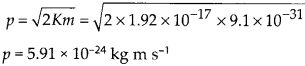(b) Speed of electron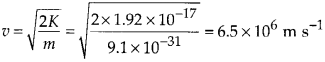(c) de-Broglie wavelength associated with electronQuestion 14.
The wavelength of light from the spectral emission line of sodium is 589 nm. Find the kinetic energy at which
(a) an electron, and
(b) a neutron, would have the same de Broglie wavelength.
Solution:
(a) Kinetic energy required by electron to have de-Broglie wavelength of 589 nm(b) Kinetic energy of neutron to have de- Broglie wavelength of 589 nmQuestion 15.
What is the de Broglie wavelength of
(a) a bullet of mass 0.040 kg travelling at the speed of 1.0 km s-1
(b) a ball of mass 0.060 kg moving at a speed of 1.0 ms-1, and
(c) a dust particle of mass 1.0 × 10-9 kg drifting with a speed of 2.2 m s-1?
Solution:
de Broglie wavelength$\lambda =\cfrac { h }{ mv }$
(a) Wavelength associated with bullet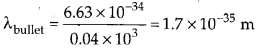(b) Wavelength associated with ball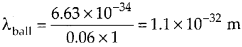(c) Wavelength associated with dust particle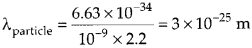Question 16.
An electron and a photon each have a wavelength of 1.00 nm. Find
(a) their momenta,
(b) the energy of the photon, and
(c) the kinetic energy of electron.
Solution:
(a) Momentum for both electron and photon will be same for same wavelength.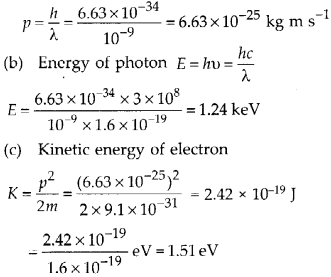Question 17.
(a) For what kinetic energy of a neutron will the associated de Broglie wavelength be 1.40 × 10-10 m?
(b) Also find the de Broglie wavelength of a neutron, in thermal equilibrium with matter having an average kinetic energy
Solution:

Question 18.
Show that the wavelength of electromagnetic radiation is equal to the de Broglie wavelength of its quantum (photon).
Solution:
For a photon, de Broglie wavelength,$\lambda =\cfrac { h }{ p } .$
For an electromagnetic radiation of frequency u and wavelength λ'(= c/v), Momentum,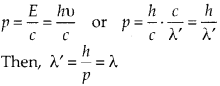Thus the wavelength of the electromagnetic radiation is the same as the de Broglie wavelength of the photon.

Question 19.
What is the de Broglie wavelength of a nitrogen molecule in air at 300 K? Assume that the molecule is moving with the root-mean-square speed of molecules at this temperature. (Atomic mass of nitrogen = 14.0076 u, K = t. 38 × 10-23 J K-1).
Solution:
Let us first calculate mass of each N2 molecule.Question 20.
(a) Estimate the speed with which electrons emitted from a heated cathode of an evacuated tube impinge on the anode maintained at a potential difference of 500 V with respect to the cathode. Ignore the small initial speeds of the electrons. The ‘specific charge’ of the electron i.e., its e/m is given to be 1.76 × 1011 C kg-1.
(b) Use the same formula you employ in (a) to obtain electron speed for an anode potential of 10 MV. Do you see what is wrong? In what way is the formula to be modified?
Solution:
(a) Energy of accelerated electronThis speed of electron is impossible. Since nothing can move with a speed greater than speed of light (c = 3 × 1o8 m s-1 The formula for kinetic energy E = 1/2 mv2 valid only for v « c. For the situation when speed v is comparable to speed of light c, we use relativistic formula. The relativistic mass is given bySubstituting the values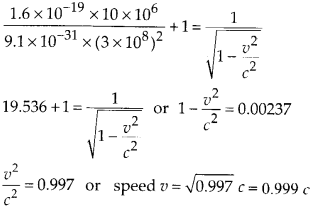Question 21.
(a) A monoenergetic electron beam with electron speed of 5.20 × 106 m s-1 is subjected to a magnetic field of 1.30 × 10-4 T, normal to the beam velocity. What is the radius of the circle traced by the beam, given e/m for electron equals 1.76 × 1011 C
kg-1.
(b) Is the formula you employ in (a) valid for calculating radius of the path of a 20 MeV electron beam? If not, in what way is it modified?
Solution:The normal magnetic field provides necessary centripetal force to the electron beam so that it can follow a circular path. Thus Force on an electron = Centripetal force due to magnetic field on an electronThe formula for radius of circular path is not valid at very high energies because such high energyQuestion 22.
An electron gun with its collector at a potential of 100 V fires out electrons in a spherical bulb containing hydrogen gas at low pressure (10-2 mm of Hg). A magnetic field of 2.83 × 10-4 T curves the path of the electrons in a circular orbit of radius 12.0 cm. Determine e/m from the data.
Solution:

Question 23.
(a) An X-ray tube produces a continuous spectrum of radiation with its short wavelength end at 0.45 A. What is the maximum energy of a photon in the radiation?
(b) From your answer to (a), guess what order of accelerating voltage (for electrons) is required in such a tube ?
Solution:
(a) Minimum wavelength corresponds to maximum energy photons.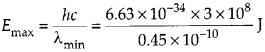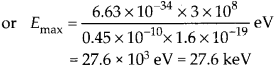(b) In order to emit photons from the surface of energy 27.6 keV, the incident electrons striking on surface should have higher energy i.e., of the order of 30 keV.

Question 24.
In an accelerator experiment on high energy collisions of electrons with positrons, a certain event is interpreted as annihilation of an electron positron pair of total energy 10.2 BeV into two y-rays of equal energy. What is the wavelength associated with each y-ray? (1 BeV = 109 eV).
Solution:
In the process of annihilation, the total energy of electron-positron pair is shared equally by both y ray photons produced. Energy of two y-rays = Energy of electron- positron pair = 10.2 BeV = 10.2 × 109 eV Energy of each y-ray photon is E = 5.1 × 109 eV = 5.1 x 109 x 1.6 x 10-19 J = 5.1 × 1.6 x 10-10 J But E = hv =$\cfrac { hc }{ \lambda }$  Hence, wavelength associated with y-ray isQuestion 25.
Estimating the following two numbers should be interesting. The first number will tell you why radio engineers do not need to worry much about ‘photons’. The second number tells you why our eye can never’eount photons’ even barely detectable light.
(a) The number of photons emitted per , second by a MW transmitter of 10 kW power emitting radio waves of length 500 m.
(b) The number of photons entering the pupil of our eye per second corresponding to the minimum intensity of white light that we humans can perceive (~ 10-10 W m-2). Take the area of the pupil ‘to be about 0.4 cm2 and the average frequency of white light to be about 6 × 1014 Hz.
Solution:
(a) Here, power of tansmitter,

exceedingly small and the number of photons emitted per second in a radio beam is enormously large. Therefore, negligible error involved in ignoring the existence of a minimum quantum of energy (photon) and treating the total energy of a radio wave as continuous.
(b) Here, area of the pupil, A = 0.4 cm2 = 0.4 × 10-4 m2, v = 6 × 1014 Hz Intensity = 10-10 W m-2 Energy of a photon is given by, E = hv = 6.63 × 10-34 × 6 × 1014 J = 4 × 10-19 J.
If n = number of photons falling per sec per unit area, the energy per unit area per sec due to these photons = total energy of n photons = n × 4 × 10-19 J nr2 Since, intensity = energy per unit area per second$10^{ -10 }=n\times 4\times { 10 }^{ -19 }$$n=\frac { 10^{ -10 } }{ 4\times 10^{ -19 } } =2.5\times 10^{ 8 }m^{ -2 }s^{ -2 }$
∴ Number of photons entering the pupil per second = n x area of the pupil = 2.5 × 108 × 0.4 × 10-4 s-1 = 104 s-1.
Though this number is not large as in part (a) above, it is large enough for us to ‘sense’ or ‘count’ the individual photons by our eye.

Question 26.
Ultraviolet light of wavelength 2271 A from a 100 W mercury source irradiates a photo-cell made of molybdenum metal. If the stopping potential is -1.3 V, estimate the work function of the metal. How would the photo-cell respond to a high intensity (~ 105 W m2) red light of wavelength 6328 A produced by a He-Ne laser?
Solution:
Let us find energy of each photon of given ultraviolet lightMaximum kinetic energy of emitted electron can be judged by stopping potential of 1.3 volt.Thus energy of red light photons is less than work function 4.17 eV, hence irrespective of any intensity, no emission will take place.

Question 27.
Monochromatic radiation of wavelength 640.2 nm (1 nm = 10-9 m) from a neon lamp irradiates photosensitive material made of caesium on tungsten. The stopping voltage is measured to be 0.54 V.The source is replaced by an iron source and its 427.2 nm line irradiates the same photo-cell. Predict the new stopping voltage.
Solution:
From the first data, work function of given photosensitive material can be calculated.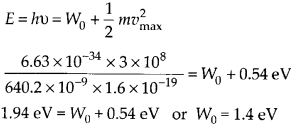Now the source is replaced by iron source which produce 427.2 nm wavelength.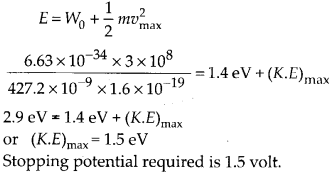Question 28.
A mercury lamp is a convenient source for studying frequency dependence of photoelectric emission, since it gives a number of spectral lines ranging from the UV to the red end of the visible spectrum. In our experiment with rubidium photo-cell, the following lines from a mercury source were used :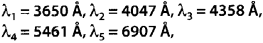The stopping voltages, respectively, were measured to be: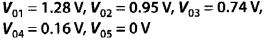Determine the value of Planck’s constant h, the threshold frequency and work function for the material.
Solution:
In order to calculate Planck’s constant ‘ll’ we need slope of the graph between cut off voltage and frequency. So, let us first calculate the frequency (u = c/λ) in each case and the table shows corresponding stopping potential.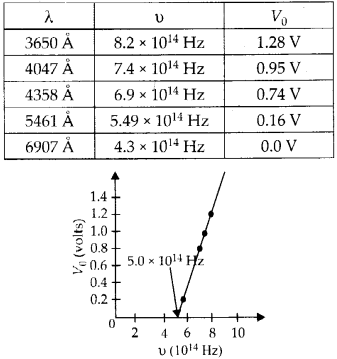V0 versus n plot shows that the first four points lie nearly on a straight line which intercepts the x-axis at threshold frequency, u0 = 5.0 × 1014 Hz. The fifth point u (= 4.3 × 1014 Hz) corresponds to u < u0, so there is no photoelectric emission and no stopping voltage is required to stop the current.
Slope of, V0 versus u graph is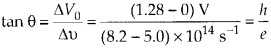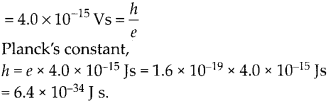(b) threshold frequency, v0 = 5.0 × 1014 J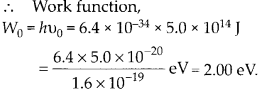Question 29.
The work function for the following metals is given:
Na: 2.75 eV; K: 2.30 eV; Mo: 4.17 eV; Ni: 5.15 eV. Which of these metals will notgive photoelectric emission for a radiation of wavelength 3300 A from a He-Cd laser placed 1 m away from the photocell? What happens if the laser is brought nearer and placed 50 cm away?
Solution:
The distance between laser source and receiver does not affect the energy of each photon incident, hence does not affect the energy of emitted photo electrons. But the reduction in distance will increase the intensity of incident light and hence number of photons. This will increase the photoelectric current. where, wavelength of incident radiation isNow, work function of Mo: 4.17 eV, Ni: 5.15 eV is more than energy of incident photon, hence these two metals will not give photoelectric emission.

Question 30.
Light of intensity 10-5 W m-2 falls on a sodium photo-cell of surface area 2 cm2. Assuming that the top 5 layers of sodium absorb the incident energy, estimate time required for photoelectric emission in the wave-picture of radiation. The work function for the metal is given to be about 2 eV. What is the implication of your answer?
Solution:
Wave picture of radiation state that incident energy is uniformly distributed among all the electrons continuously. Let us first calculate the total number of recipient electrons in-5 layers of sodium. Consider each sodium atom has one electron free as conduction electron. Effective atomic area = 10-20 m2 Number of conduction electrons in 5 layers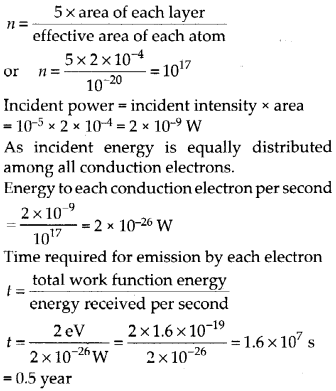Where experimental observation shows that emission of photo electrons is instantaneous = 10-9 sec Thus wave picture fails to explain photoelectric effect.

Question 31.
Crystal diffraction experiments can be perfor- med using X-rays, or electrons accelerated through appropriate voltage. Which probe has greater energy? An X-ray photon or the electron? (For quantitative comparison, take the wavelength of the probe equal to 1 A, which is of the order of inter-atomic spacing in the lattice) (me = 9.11 × 10-31 kg).
Solution:
Order of interatomic spacing is 1 A in the crystal lattice. So, for diffraction to take place the wavelength should be of the same . order. For X-ray photon (energy for wavelength of 1 A)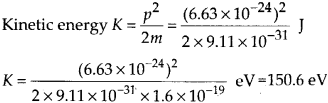Thus in order to produce same wavelength X-ray photon should have higher energy than electron.

Question 32.
(a) Obtain the de Broglie wavelength of a neutron of kinetic energy 150 eV. An electron beam of this energy is suitable for crystal diffraction experiments. Would a neutron beam of the same energy be equally suitable? Explain. (mn = 1.675 × 10-27 kg)
(b) Obtain the de Broglie wavelength associated with thermal neutrons at room temperature (27°C). Hence explain why a fast neutron beam needs to be thermalised with the environment before it can be used for neutron diffraction experiments.
Solution:
(a) Let us first calculate the wavelength of matter wave associated with neutron of kinetic energy 150 eV.As the inter atomic spacing (1 A= 10-10 m) is about hundred times greater than this wavelength, so a neutron beam of 150 eV energy is not suitable for diffraction experiments.

(b) Average kinetic energy of a neutron at absolute temperature T is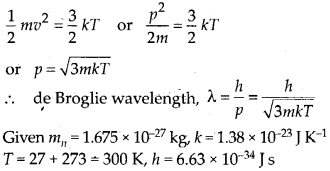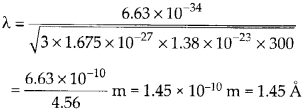As this wavelength is comparable to inter atomic spacing (= 1 A) in a crystal, so thermal neutrons can be used for diffraction experiments. So high energy neutron beam should be first thermalised before using it for diffraction.

Question 33.
An electron microscope uses electrons accelerated by a voltage of 50 kV. Determine the de-Broglie wavelength associated with the electrons. If other factors (such as numerical aperture etc.) are taken to be roughly the same, how does the resolving power of an electron microscope compare with that of an optical microscope which uses yellow light?
Solution:
K.E. of an electron, accelerated by voltage of 50 kV.Thus, the resolving power of an electron microscope is about 103 times greater than that of an optical microscope.

Question 34.
The wavelength of a probe is roughly a measure of the size of a structure that it can probe in some detail. The quark structure of protons and neutrons appears at the minute length scale of 10-15 m or less. This structure was first probed in early 1970’s using high energy electron beam produced by a linear accelerator at Stand ford, USA. Guess what might have been the order of energy of these electron beams. (Rest mass energy of electron = 0.511 M eV).
Solution:
Momentum of electron associated with given wavelength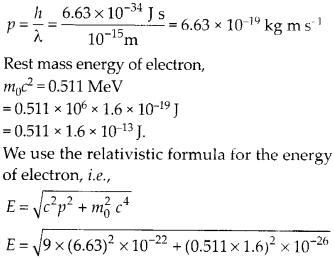Second term showing rest mass energy is negligible. Energy, E = 1.989 × 10-10 J = 1.24 BeV Thus, energies acquired by electron from the given accelerator must have been of the order of a few BeV.

Question 35.
Find the typical de-Broglie wavelength associated with a He atom in helium gas at room temperature (27°C) and 1 atm pressure; and compare it with the mean separation between two atoms under these conditions.
Solution:
Let us first find mass ‘in’ of each helium atom.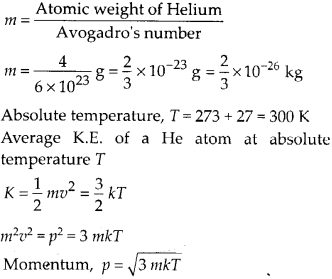Wavelength of the wave associated with He atom at room.temperature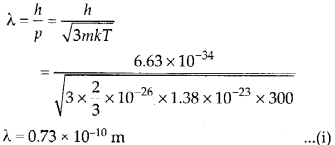Now let us find mean separation between He atoms. mean separation,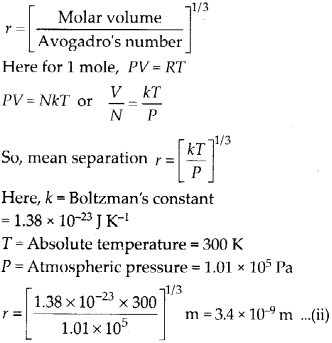Comparing the wavelength ‘X’ with mean separation V, it can be observed that separa¬tion is larger than

Question 36.
Compute the typical de Broglie wavelength of an electron in a metal at 27° C and compare it with the mean separation between two electrons in a metal which is given to be about 2 × 10-10 m.
Solution:
Considering free electrons as gas. Kinetic energy at temperature TGiven that mean separation between two electrons is about 2 × 10-10 m.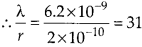So, de-Broglie wavelength is much greater than the electron separation.

Question 37.
(a) Quarks inside protons and neutrons are thought to carry fractional charges [(+ 2/3)e ; {—1/3)e]. Why do they not show up in Millikan’s oil-drop experiment?
(b) What is so special about the combination e/m? Why do we not simply talk of e and m separately?
(c) Why should gases be insulators at ordinary pressures and start conducting at very low pressure?
(d) Every metal has a definite work function. Why do all photo electrons not come out with the same energy if incident radiation is monochromatic? Why is there an energy distribution of photo electrons?
(e) The energy and momentum of an electron are related to the frequency and wavelength of the associated matter wave by the relations: E = hv, p = h/λ
But while the value of X is physically significant, the value of u (and therefore, the value of phase speed uλ) has no physical significance. Why?
Solution:
(a) The quarks having fractional charges are thought to be confined within a proton and a neutron. These quarks are bound by forces. These forces become stronger when the quarks are tried to be pulled apart. That is why, the quarks always remain together. It is due to this reason that though fractional charges do exist in nature but the observable charges are always integral multiple of charge of electron.

(b)
The motion of electron in the electric and magnetic field is related with the basic equations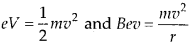All these equations involve e and m together, i.e., there is no equation in which e or m occurring alone. As a result of it, we study e/m of electron and do not talk of e and m separately for an electron.

(c)
At ordinary pressures a few positive ions and electrons produced by the ionisation of the gas molecules by energetic rays (like X-rays, y-rays, cosmic rays etc. coming from outer space and entering the earth’s atmosphere) are not able to reach their respective electrodes, even at high voltages, due to their frequent collisions with gas molecules and recombinations. That is why the gases at ordinary pressures are insulators.
At low pressures, the density of the gas decreases, the mean free path of the gas molecules become large. Now under the effect of external high voltage, the ions acquire sufficient energy before they collide with molecules causing further ionisation. Due to it, the number of ions in the gas increases and it becomes a conductor.

(d)
By work function of a metal, we mean the minimum energy required for the electron
in the highest level of conduction band to get out of the metal. Since all the electrons in the metal do not belong to that level but they occupy a continuous band of levels, therefore, for the given incident radiation, electrons knocked off from different levels come out with different energies.

(e)
de broglie wavelength associated with the moving particle is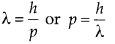Energy of the wave is E= hv =$\cfrac { hc }{ \lambda }$ Energy of moving particleAll Chapter NCERT Solutions For Class12 Physics

—————————————————————————–

All Subject NCERT Solutions For Class12

*************************************************

Remark:

I think you got complete solutions for this chapter. If You have any queries regarding this chapter, please comment on the below section our subject teacher will answer you. We tried our best to give complete solutions so you got good marks in your exam.

If these solutions have helped you, you can also share Careerkundali.in to your friends.

Best of Luck!!